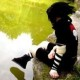# 48 နာရီအတွင်းတဲ ့ 1 လာတော့မယ်

N Indian Ocean: Storm Alert issued at 18 May, 2010 0:00 GMT

Tropical Storm ONE (01B) is forecast to strike land to the following likelihood(s) at the given lead time(s):

India

probability for CAT 1 or above is 20% in about 48 hours
probability for TS is 60% in about 48 hours

Machilipatnam (16.2 N, 81.2 E)
probability for CAT 1 or above is 20% in about 72 hours
probability for TS is 55% in about 48 hours
Kavali (15.0 N, 80.0 E)
probability for CAT 1 or above is 15% in about 48 hours
probability for TS is 55% in about 48 hours
Nellore (14.4 N, 80.0 E)
probability for CAT 1 or above is 15% in about 48 hours
probability for TS is 55% in about 48 hours
probability for CAT 1 or above is 20% in about 72 hours

probability for TS is 40% in about 48 hours

Vishakhapatnam (17.8 N, 83.3 E)

probability for CAT 1 or above is 15% in about 72 hours

probability for TS is 35% in about 72 hours
probability for CAT 1 or above is 10% in about 96 hours

probability for TS is 30% in about 72 hours

Brahmapur (19.3 N, 84.9 E)
probability for CAT 1 or above is 10% in about 96 hours

probability for TS is 20% in about 72 hours

#### One comment

•May 18, 2010 at 12:00 pm

May be India will more attacked according to your data.